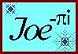Cross Vault Sketches, Notes and Links
Study of an Irregular Cross Vault

 Cross Vault Purlin Angle Calculator
Cross Vault Rise =
Plan Angle between Centerlines =
Main Vault Width =
 Measured from the Center of the Main Vault
Dimension to Framing Point =
 MAIN VAULT ELLIPSE
Plan Angle =
Foci from Center ± =
Rise to Framing Point =
Slope at Framing Point =
Pitch Angle at Framing Point =
 Purlin Face tangent to the Curve
Angle on the Stick =
Saw Miter Angle =
 Purlin Face perpendicular to the Curve
Angle on the Stick =
Saw Miter Angle =
 VALLEY ELLIPSE
Width =
Foci from Center ± =
Center to Framing Point =
Slope at Framing Point =
Pitch Angle at Framing Point =
Plan Angle =
Foci from Center ± =
Center to Framing Point =
Slope at Framing Point =
Pitch Angle at Framing Point =
 Purlin Face tangent to the Curve
Angle on the Stick =
Saw Miter Angle =